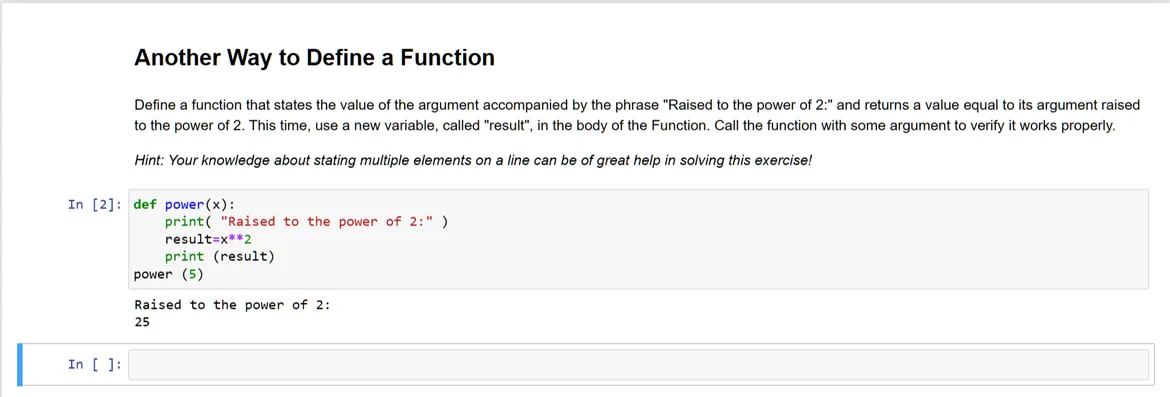29 Aug 2023

Posted on:

12 Aug 2023

0

# Resolved:Print function returns calculated output

In my case print function can also provide the calculated result. It seems like print and return are interchangeable though return can be used once in a block whereas print can be used multiple times.Instructor
Posted on:

29 Aug 2023

0

Hi Fazley!
Thanks for reaching out.

We use return when we want to return some result. But nothing is shown on console. To have the result in the console, we use print. Let me show you with an example. If you have:

`def multiplication_by_2(x):   `
`    print(x*2)`
We call the function with an argument and it works! Fantastic! But if you want to use return we should:

`def multiplication_by_2(x):      `
`    return x*2`
What we have here? The same function but instead of print we have return. So if we invoke our function with argument 5 -> `multiplication_by_2(5)`, the result will be shown in the console but from the console of Jupyter Notebook, not from Python. So we have to use print like this:

`def multiplication_by_2(x):   `
`    return x*2  `
`result=multiplication_by_2(5)`
`print(result)`
When this code is executed it shows `10`.

To sum up, when using print in a function, we invoke the function and it works. When using return we invoke the function and store the result in a variable which we then print with print() function.

Hope this helps.
Best,
Tsvetelin